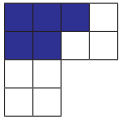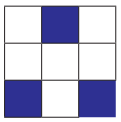Students can download 5th Maths Term 3 Chapter 6 Fractions 6.1 Questions and Answers, Notes, Samacheer Kalvi 5th Maths Guide Pdf helps you to revise the complete Tamilnadu State Board New Syllabus, helps students complete homework assignments and to score high marks in board exams.

## Tamilnadu Samacheer Kalvi 5th Maths Solutions Term 3 Chapter 6 Fractions 6.1

Question 1.
Write the fraction shown by the shaded parts.
(i)$$\frac { 5 }{ 12 }$$(ii)$$\frac { 2 }{ 6 }$$

(iii)$$\frac { 3 }{ 9 }$$Question 2.
Write the fractions for the following and find the numerator and the denominator.
(i) Sheeki got 12 marks out of 20 marks in science.
$$\frac { 12 }{ 20 }$$; Numerator = 12; Denominator = 20

(ii) Six fruits are rotten out of forty fruits in a basket.
$$\frac { 6 }{ 40 }$$; Numerator = 6; Denominator = 40

(iii) In a colony of 50 houses. 17 houses are not occupied.
$$\frac { 17 }{ 50 }$$; Numerator = 17; Denominator = 50Question 3.
Find out which is greater in the following fractions.
(i) $$\frac { 5 }{ 12 }$$ and $$\frac { 7 }{ 12 }$$
(ii) $$\frac { 22 }{ 48 }$$ and $$\frac { 17 }{ 48 }$$
(iii) $$\frac { 11 }{ 56 }$$ and $$\frac { 27 }{ 56 }$$
(i) $$\frac { 5 }{ 12 }$$ and $$\frac { 7 }{ 12 }$$
5 < 7
∴ $$\frac { 5 }{ 12 }$$ < $$\frac { 7 }{ 12 }$$
$$\frac { 7 }{ 12 }$$ is greater

(ii) $$\frac { 22 }{ 48 }$$ and $$\frac { 17 }{ 48 }$$
22 > 17
∴ $$\frac { 22 }{ 48 }$$ > $$\frac { 17 }{ 48 }$$
$$\frac { 22 }{ 48 }$$ is greater.(iii) $$\frac { 11 }{ 56 }$$ and $$\frac { 27 }{ 56 }$$
11 < 27
∴ $$\frac { 11 }{ 56 }$$ < $$\frac { 27 }{ 56 }$$
$$\frac { 27 }{ 56 }$$
11 < 27 is greater

Question 4.
Which is smaller in the following fraction?
(i) $$\frac { 10 }{ 42 }$$ and $$\frac { 21 }{ 42 }$$
(ii) $$\frac { 31 }{ 37 }$$ and $$\frac { 15 }{ 37 }$$
(i) $$\frac { 10 }{ 42 }$$ and $$\frac { 21 }{ 42 }$$
$$\frac { 10 }{ 42 }$$ < $$\frac { 21 }{ 42 }$$
$$\frac { 10 }{ 42 }$$ is smaller(ii) $$\frac { 31 }{ 37 }$$ and $$\frac { 15 }{ 37 }$$
$$\frac { 31 }{ 37 }$$ < $$\frac { 15 }{ 37 }$$
$$\frac { 15 }{ 37 }$$ is smaller